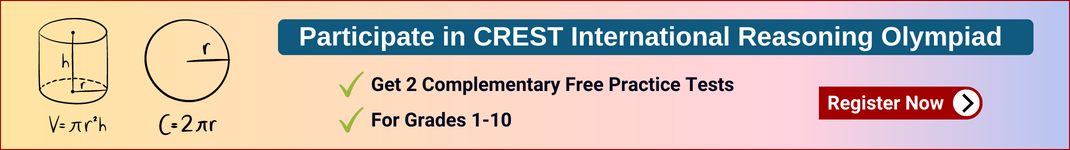# Mental Maths Worksheet for Class 8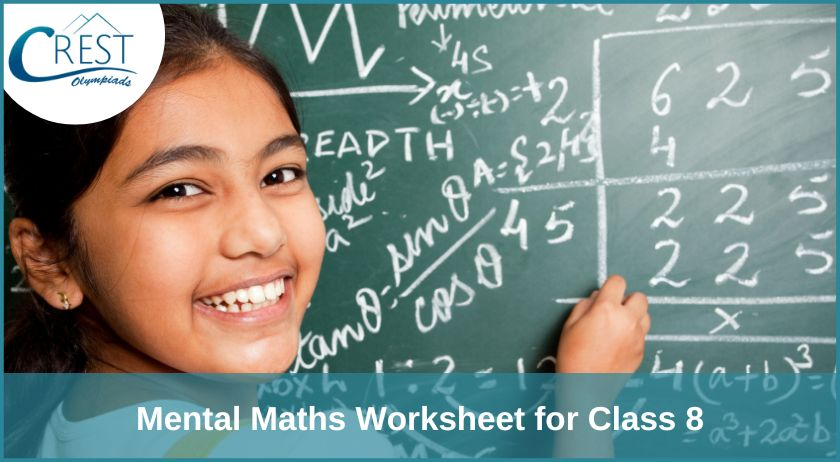Mental Maths worksheet for class 8 is a practice sheet designed to help students develop their mental arithmetic skills. The worksheet contains a series of math problems. The purpose of this worksheet is to enhance students' ability to perform quick calculations in their minds and increase their overall speed and accuracy in solving Math problems.

Mental Maths worksheet for class 8 is an excellent way to reinforce mathematical concepts learned in class, as well as help students develop their problem-solving and critical thinking skills. This can also be used as a pre-assessment tool to gauge students' understanding of a particular topic before moving on to more advanced concepts.

Students can benefit greatly from regular practice of Mental Maths questions for class 8. Hence, we recommend students to practice following questions.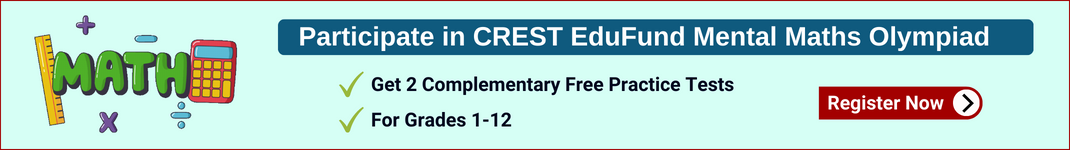## Mental Maths Worksheet for Class 8

1. What is the equivalent fraction for 0.75?

a) 3/4
b) 4/3
c) 7/5
d) 5/7

2. What is the value of the?
1, 4, 7, 10, 13

a) 16
b) 14
c) 15
d) 19

3. What is the slope of the line y = 2x + 1?

a) 2
b) 3
c) 4
d) 5

4. What is the formula for finding the volume of a cylinder?

a) πr2h
b) πr3h
c) πrh2
d) πr2h2

5. What is the median of the following set of numbers: 3, 4, 7, 8, 9?

a) 6
b) 7
c) 8
d) 9

6. What is the probability of rolling a 4 on a standard dice?

a) 1/6
b) 1/5
c) 1/4
d) 1/3

7. What is the length of a rectangular room measuring 7 meters by 4 meters?

a) 11 meters
b) 28 meters
c) 31 meters
d) 35 meters

8. What is the volume (capacity) of a rectangular prism with a length of 10 cm, a width of 5 cm, and a height of 2 cm?

a) 100 cm3
b) 60 cm3
c) 20 cm3
d) 10 cm3

9. What is the area of a triangle with a base of 8 cm and a height of 5 cm?

a) 20 cm2
b) 40 cm2
c) 30 cm2
d) 50 cm2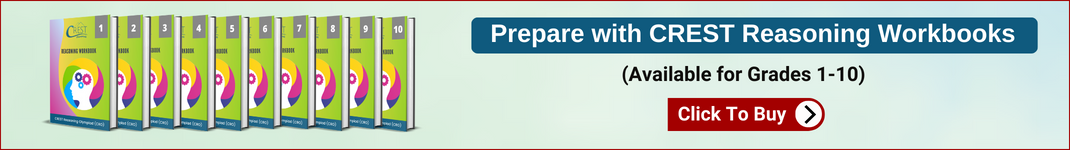### Solve

1. What is the volume (capacity) of a rectangular prism with a length of 10 cm, a width of 6 cm, and a height of 5 cm?

a) 120 cm³
b) 350 cm³
c) 300 cm³
d) 700 cm³

2. What is the area of a circle with a radius of 6 cm?

a) 113.1 cm²
b) 36 cm²
c) 72 cm²
d) 28.26 cm²

3. What is the volume of a cylinder with a height of 8 cm and a radius of 4 cm?

a) 101.13 cm³
b) 402.12 cm³
c) 40.45 cm³
d) 80.90 cm³

4. Which of the following shapes is a sphere?

a) Cube
b) Cylinder
c) Rectangular prism
d) Ball

5. How many sides does a triangle have?

a) 4
b) 5
c) 3
d) 6

6. What is the product of 5/6 and 4/5?

a) 1/3
b) 1/2
c) 4/5
d) 2/3

7. What is the sum of 4/5 and 2/5?

a) 6/5
b) 1/2
c) 3/5
d) 2/3

1. c) 300 cm3
2. a) 113.1 cm²
3. b) 402.12 cm³
4. d) Ball
5. c) 3
6. d) 2/3
7. a) 6/5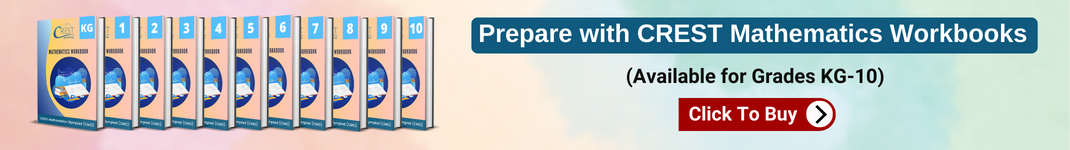## Mental Maths Worksheet PDF for Class 8

Mental Maths worksheet PDF for class 8 is readily available to download and that too is free. This worksheet is a great way to practice and develop Mental Maths skills by practicing given questions daily. Also, answer key for the questions is provided.

This worksheet carries a structured approach which is particularly important for students who may find Mental Maths challenging, as it allows them to work through the exercises at their own pace. With the smooth accessibility, one can download the pdf for class 8 Mental Maths worksheet by the given link below: